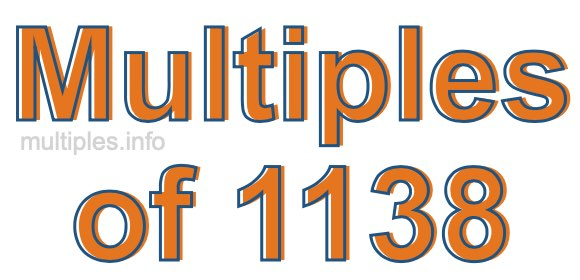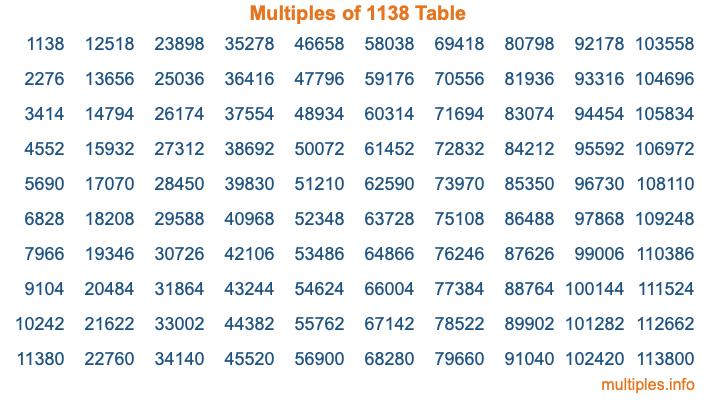Multiples of 1138Welcome to the Multiples of 1138 page. Here we will first teach you everything you will ever need to know about the multiples of 1138, and then give you a study guide summary of everything we taught you to make sure you remember it all. Use this page to look up facts and learn information about the multiples of 1138. This page will make you a multiples of one thousand one hundred thirty-eight expert!

Definition of Multiples of 1138
Multiples of 1138 are all the numbers that when divided by 1138 equal an integer. Each of the multiples of 1138 are called a multiple. A multiple of 1138 is created by multiplying 1138 by an integer.

Therefore, to create a list of multiples of 1138, you start with 1 multiplied by 1138, then 2 multiplied by 1138, then 3 multiplied by 1138, and so on for as long as you want. Thus, the list of the first five multiples of 1138 is 1138, 2276, 3414, 4552, and 5690. To see a larger list of multiples of 1138, see the printable image of Multiples of 1138 further down on this page. We also have a category where you can choose any nth multiple of 1138.

Multiples of 1138 Checker
The Multiples of 1138 Checker below checks to see if any number of your choice is a multiple of 1138. In other words, it checks to see if there is any number (integer) that when multiplied by 1138 will equal your number. To do that, we divide your number by 1138. If the the quotient is an integer, then your number is a multiple of 1138.

Is  a multiple of 1138?

Least Common Multiple of 1138 and ...
A Least Common Multiple (LCM) is the lowest multiple that two or more numbers have in common. This is also called the smallest common multiple or lowest common multiple and is useful to know when you are adding our subtracting fractions. Enter one or more numbers below (1138 is already entered) to find the LCM.

Check out our LCM Calculator if you need more details about the Least Common Multiple or if you need the LCM for different numbers for adding and subtraction fractions.

nth Multiple of 1138
As we stated above, 1138 is the first multiple of 1138, 2276 is the second multiple of 1138, 3414 is the third multiple of 1138, and so on. Enter a number below to find the nth multiple of 1138.

th multiple of 1138

Multiples of 1138 vs Factors of 1138
1138 is a multiple of 1138 and a factor of 1138, but that is where the similarities end. All postive multiples of 1138 are 1138 or greater than 1138. All positive factors of 1138 are 1138 or less than 1138.

Below is the beginning list of multiples of 1138 and the factors of 1138 so you can compare:

Multiples of 1138: 1138, 2276, 3414, 4552, 5690, etc.

Factors of 1138: 1, 2, 569, 1138

As you can see, the multiples of 1138 are all the numbers that you can divide by 1138 to get a whole number. The factors of 1138, on the other hand, are all the whole numbers that you can multiply by another whole number to get 1138.

It's also interesting to note that if a number (x) is a factor of 1138, then 1138 will also be a multiple of that number (x).

Multiples of 1138 vs Divisors of 1138
The divisors of 1138 are all the integers that 1138 can be divided by evenly. Below is a list of the divisors of 1138.

Divisors of 1138: 1, 2, 569, 1138

The interesting thing to note here is that if you take any multiple of 1138 and divide it by a divisor of 1138, you will see that the quotient is an integer.

Multiples of 1138 Table
Below is an image of the first 100 multiples of 1138 in a table. The table is in chronological order, column by column. The first column has the first ten multiples of 1138, the second column has the next ten multiples of 1138, and so on.The Multiples of 1138 Table is also referred to as the 1138 Times Table or Times Table of 1138. You are welcome to print out our table for your studies.

Negative Multiples of 1138
Although not often discussed or needed in math, it is worth mentioning that you can make a list of negative multiples of 1138 by multiplying 1138 by -1, then by -2, then by -3, and so on, to get the following list of negative multiples of 1138:

-1138, -2276, -3414, -4552, -5690, etc.

Multiples of 1138 Summary
Below is a summary of important Multiples of 1138 facts that we have discussed on this page. To retain the knowledge on this page, we recommend that you read through the summary and explain to yourself or a study partner why they hold true.

There are an infinite number of multiples of 1138.

A multiple of 1138 divided by 1138 will equal a whole number.

1138 divided by a factor of 1138 equals a divisor of 1138.

The nth multiple of 1138 is n times 1138.

The largest factor of 1138 is equal to the first positive multiple of 1138.

1138 is a multiple of every factor of 1138.

1138 is a multiple of 1138.

A multiple of 1138 divided by a divisor of 1138 equals an integer.

1138 divided by a divisor of 1138 equals a factor of 1138.

Any integer times 1138 will equal a multiple of 1138.

Multiples of a Number
Here you can get the multiples of another number, all with the same attention to detail as we did for multiples of 1138 on this page.

Multiples of
Multiples of 1139
Did you find our page about multiples of one thousand one hundred thirty-eight educational? Do you want more knowledge? Check out the multiples of the next number on our list!